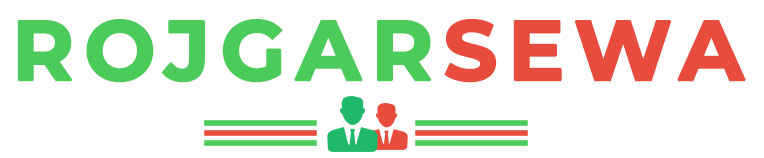## Aptitude :: SBI PO

1.

If x, y and z are odd integers then which of the following is necessarily false?

 A. xyz is odd B. z(x-y) is even C. x(x-y)(z+y) is even D. (x-y-z)(x+z) is odd
1.

HCF of two number is 15 and their LCM is 180. If their sum is 105, then the numbers are

 A. 45 and 60 B. 30 and 75 C. 35 and 70 D. 40 and 65
1.

Consider 3 consecutive prime integers. Twice the first integer is 5 more than the third integer and second is 4 less than the third integer. Sum of the integers is 41. Find the smallest integer among the three.

 A. 11 B. 13 C. 7 D. 5
1.

Number Systems

 A. B. C. D.